Markets
Futures
Earn
More
Cancel
FAQAccount FunctionsTutorialBinance Fan TokenBinance EarnCrypto Deposit/Withdrawal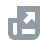Crypto Derivatives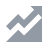FinanceAPISecurityOther Topics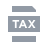Tax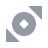Binance Convert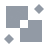NFTVIP
Home
Support Center
FAQ
Tiered Leverage Function on Isolated Margin

# Tiered Leverage Function on Isolated Margin

2020-07-02 09:52

## 1. Introduction

Using Tiered Leverage, the system automatically adjusts the maximum leverage based on the user’s borrowed fund amount. This means that the leverage for an Isolated trading pair is dynamic. So, the corresponding initial risk ratio will vary. With Tiered Leverage, there are 10 tiers for 10x Isolated Margin and 5 tiers for 5x and 3x. The same margin call and liquidation risk ratios will apply to all tiers under the same leverage level on Isolated Margin trading pairs, as shown in the tables below.

## 2. Tiered Leverage Risk Parameters

• 10-level tiered leverage supports up to 10x leverage on Isolated Trading pairs:
• 5-level tiered leverage supports up to 5x leverage on Isolated Trading pairs:
• 5-level tiered leverage supports up to 3x leverage on Isolated Trading pairs:

## 3. Borrowing Impact

• The greater the amount of funds borrowed, the higher the tiered leverage level; the lower the maximum leverage level, the higher the initial risk ratio.
• The smaller the amount of funds borrowed, the lower the tiered leverage level; the higher the maximum leverage level, the lower the initial risk ratio.
• If the user simultaneously borrows the base currency and the quote currency, the system calculates the new levels separately and the tier will be determined based on whichever is higher.
Using BTC/USDT as an example, assume the Tiered Leverage as shown in the following table:
• Assuming the user borrowed 15 BTC and 250,000 USDT on Isolated Margin:
• If the borrowed BTC tier is 2 and the borrowed USDT tier is 4, the higher tier will be used (tier 4);
• The maximum leverage is 7.36 and the corresponding liquidation risk ratio is 1.05.
• Borrowing for VIP users and sub-accounts
• For VIP users and sub-accounts, the system will automatically match the corresponding tiered leverage level based on the amount borrowed.

## 4. Isolated Liquidation

When the Margin Level hits the liquidation risk ratio, it will trigger liquidation. Your position will be partially liquidated and liquidation will stop when the Margin Level reaches the Margin Call Ratio. In extreme cases, all positions will be liquidated.
For example, User A borrows 250,000 USDT in the BTCUSDT Isolated Margin account that supports up to 10x leverage. The Margin Call Ratio is 1.1 and the Liquidation Risk Ratio is 1.05. When the Margin Level ≤ 1.05, User A’s collateral assets will be liquidated to pay back the outstanding loan amount and interest. In most cases, the liquidation process will stop when the Margin Level is restored to 1.1 (Margin Call Ratio) or higher.

## 5. How to Check Tiered Leverage

You can view the maximum leverage for Isolated Margin from [Wallet] - [Margin] - [Isolated].
Alternatively, you may view the tier information on the Borrow/Repay pop-up.
For more details, please visit Isolated Margin Tier Data.